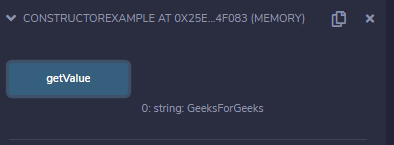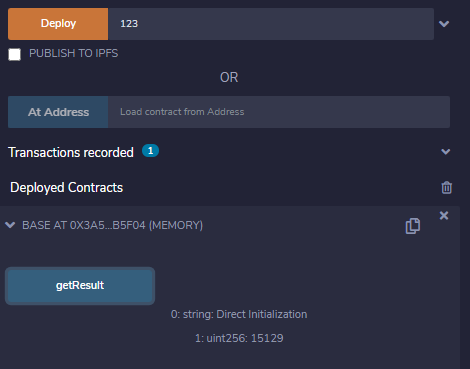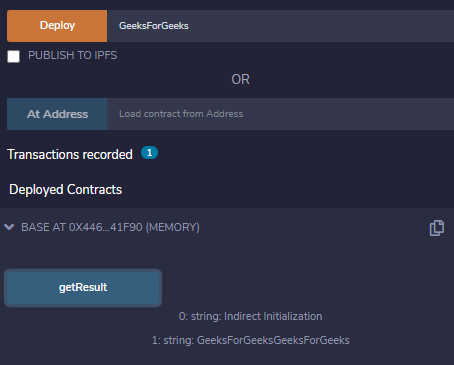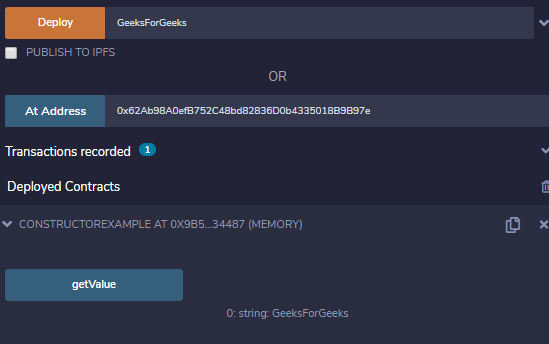Open In App
Related Articles

# Solidity – Constructors

A constructor is a special method in any object-oriented programming language which gets called whenever an object of a class is initialized. It is totally different in case of Solidity, Solidity provides a constructor declaration inside the smart contract and it invokes only once when the contract is deployed and is used to initialize the contract state. A default constructor is created by the compiler if there is no explicitly defined constructor.

### Creating a constructor

A Constructor is defined using a constructor keyword without any function name followed by an access modifier. It’s an optional function which initializes state variables of the contract. A constructor can be either internal or public, an internal constructor marks contract as abstract.

Syntax:

```constructor() <Access Modifier> {
} ```

Example: In the below example, in the contract constructorExample, a constructor is created to initialize the state variable str.

## Solidity

 `// Solidity program to demonstrate``// creating a constructor``pragma solidity ^0.5.0;       ``        ` `// Creating a contract``contract` `constructorExample {       ``        ` `    ``// Declaring state variable``    ``string str;       ``            ` `    ``// Creating a constructor``    ``// to set value of 'str'``    ``constructor() ``public` `{                 ``        ``str = ``"GeeksForGeeks"``;       ``    ``}       ``     ` `    ``// Defining function to``    ``// return the value of 'str' ``    ``function getValue(``    ``) ``public` `view returns (``      ``string memory) {       ``        ``return` `str;       ``    ``}     ``}`

Output :### Constructor in Inheritance

In case if a constructor is not defined then the default constructor is called, but if the constructor is defined in a parent contract and having some arguments then the child contract should also provide the required parameters to the constructor. If the child contract is not passing any parameter to the parent’s constructor the child contract will become an abstract contract. There are two ways of calling a parent contract’s constructor:

1. Direct Initialization: In the below example, the direct initialization method is used to initialize the constructor of the parent class.

## Solidity

 `// Solidity program to demonstrate``// Constructor in Inheritance``pragma solidity ^0.5.0;` `// Creating a contract``contract` `Base {` `   ``// Declaring variable``   ``uint data;` `   ``// Defining a constructor``   ``constructor(uint _data) ``public` `{``      ``data = _data;  ``   ``}` `   ``// Defining function``   ``function Print(``   ``) ``public`  `returns(string memory){``       ``return` `"Direct Initialization"``;``   ``}` `}` `// Child contract inheriting``// the parent contract 'Base'``contract` `Derived is Base(2){` `    ``// Defining a constructor``    ``constructor() ``public` `{}` `   ``// Defining function to access``   ``// variable of parent contract``   ``function getData(``   ``) external returns(uint){``       ``uint result = data ** 2;``       ``return` `result;``   ``}``}` `// Caller contract``contract` `caller{` `   ``// Creating an object of child contract``    ``Derived c = ``new` `Derived();` `   ``// Accessing functions of parent``   ``// and child contract using``   ``// object of child contract``    ``function getResult() ``public` `returns(uint){``        ``c.Print();``        ``return` `c.getData();``    ``}``}`

Output :2. Indirect Initialization: In the below example, the indirect initialization using Base(string(abi.encodePacked(_info, _info))) is done to initialize the constructor of the base class.

## Solidity

 `// Solidity program to demonstrate``// Indirect Initialization``pragma solidity ^0.5.0;` `// Creating a contract``contract` `Base {` `   ``// Declaring state variable``   ``string str;` `   ``// Defining a constructor``   ``constructor(``     ``string memory _str) ``public` `{``      ``str = _str;  ``   ``}` `   ``// Defining a function``   ``function Print(``   ``) ``public` `returns(string memory){``       ``return` `"Indirect Initialization"``;``   ``}``}` `// Child contract inheriting``// parent contract 'Base'``contract` `Derived is Base {` `   ``// Defining a constructor``   ``constructor(``     ``string memory _info) Base(``     ``string(abi.encodePacked(``       ``_info, _info))) ``public` `{}` `   ``// Defining function to``   ``// return value of parent``   ``// contract variable 'str'``   ``function getStr(``   ``) ``public` `view returns(string memory){``       ``return` `str;``   ``}``}` `// Caller contract``contract` `caller {` `    ``// Creating an object of``    ``// child contract``    ``Derived c``      ``= ``new` `Derived(``"GeeksForGeeks"``);` `    ``//Defining a function to access``    ``// functions of the parent``    ``//contract and child contract``    ``function getResult() ``public` `view{``        ``c.Print();``        ``c.getStr();``    ``}``}`

Output :### Need of Constructors

Constructors are very useful in a smart contract, a parameter value can be defined at the run time and can also restrict the method call. Constructor overloading is not supported in Solidity, it only allows one constructor at a time.

Example: In the below example, the contract constructorExample consists of a constructor to demonstrate the need for the constructor.

## Solidity

 `// Solidity program to demonstrate``// Need of constructors``pragma solidity ^0.5.0;       ``        ` `// Creating a contract``contract` `constructorExample {       ``        ` `    ``// Declaring state variable``    ``string str;       ``    ``address ``private` `owner``      ``= 0x62Ab98A0efB752C48bd82836D0b4335018B9B97e;` `    ``// Defining constructor``    ``constructor(string memory string) ``public` `{                 ``        ``if``(msg.sender == owner){   ``            ``str = string;   ``        ``}       ``    ``}       ``    ` `    ``// Defining function to``    ``// return value of 'str'  ``    ``function getValue() ``public` `view returns (``      ``string memory) {       ``        ``return` `str;       ``    ``}     ``}`

Output :